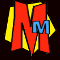# The Most Pathetic Fact in Maths

#### ☺ Some visitors even sent their own facts which we've included here. Do you have a pathetic number fact that we should include? If so please let us know! The best ones will appear here.

##### Curtis Clewett says that a number made up of a 1 followed by only 0s has as many factors as digits squared. For example 10 has 2 digits which makes 22=4 factors (and they are 1,2,5 and 10). 1,000,000 has 7 digits and so has 49 factors! Ridhan Garware found that if you look at the answers in the 8 times table, starting 8,16,24,32 etc, you work out the digital roots of each one and you get the numbers 8,7,6,5,4,3,2,1 then 9,8,7,6 etc.. and it keeps repeating! (Digital Root means you add the digits until you get a single digit. So DR of 24 = 2+4 = 6. DR of 64 = 6+4=10 then 1+0 = 1) Twitter told us that 55 x 44 x 33 x 22 x 11 = 86,400,000 = the number of milliseconds in one day! Nishanth Matthew tells us that 10! (which is 10x9x8x7x6x5x4x3x2x1 ) is the exact number of seconds in six weeks! AL enlightened our lives by telling us that TWENTY NINE is the only number that is written with as many strokes as its numerical value! (You need to write Y with 3 strokes) Gareth Latty tells us that there are 169,518,829,100,544,000,000,000,000,000 ways to play the first ten moves in a game of chess! Matthew Sheeran says that 3,608,528,850,368,400,786,036,725 has 25 digits and divides by 25. BUT... it you just take the first n digits, the result will divide by n. For example, 360852 are the first 6 digits and 360852 divides by 6. Charles Symons tells us that he likes the 1729 fact - it's the lowest number that can be expressed as the sum of two cubes in two different ways! In other words: 1729= 93+ 103 OR 13+ 123 Philip Davies says that an "octillion" is the lowest positive number to contain a letter "c". How mind numbingly useless - we salute you Philip! However a visitor called Peter pointed out that one octillionth is smaller... Philip Davies (again) says that "ONE" is the only number in the English language to have its letters in reverse alphabetical order. Sylvan Golden says that the biggest number you can make with three digits and any operators is 9 to the power of 9 to the power of 9. As 99 = 387420489, the final number is 9387420489 = er...well actually as it has about 200 million digits we won't bother writing it out! The PURE MATHEMATICIANS say that you can make an even bigger number with three digits and operators by having 9! to the power of 9! to the power of 9! (9!=9x8x7x6x5x4x3x2x1). ARGHHHH! HU YI JIE from Singapore has this peculiar sum: TWELVE PLUS ONE is an anagram of ELEVEN PLUS TWO. HU YI JIE (again) says 183 = 5832 and 184 = 104976 and that uses all the digits 0-9 (just like the "69" fact above). THE BAD HAIR BEAR tells us that 111,111,111 x 111,111,111 = 12,345,678,987,654,321. SITA tells us that the Spanish word for five is "cinco" and it's the only Spanish number to have the same number of letters in its name. (See fact 17 above) MENGQI LI from China tells us that 1 ÷ 14 = 0.0714285714285714285... and 7,14, and 28 are factors and multiples of 14 and the 5 tells you how many digits 71428 has before they repeat! BEEP from Ipswich tells us if you want to add all the numbers 1-10 , you just divide the 10 by 2 then write the answer out twice=55. Also all the numbers 1-100 is 100/2 written twice i.e. 5050. This also works for 1-1000, 1-10000, 1-100000 etc! RIDWAN says that 144 is the twelfth number in the Fibonici series 144 and it is also twelve squared! ALEX JEFFREYS tells us that 12 + 22 + 32 + 42 + 52 +... +222 + 232 + 242 = 702 Teacher JANET BATTY tells us that 1274953680 has two odd things about it: it uses all the digits 0-9 AND you can divide it exactly by any number from 1-16. Nice one Janet. MATHS ED tells us that by the end of the 9th day of the 9th month (which is 9th September) the number of minutes that have passed in the year is exactly 9! in other words 9x8x7x6x5x4x3x2x1 = 362,880.To the "NUMBERS" book